# Skip Counting By 2

Skip Counting By 2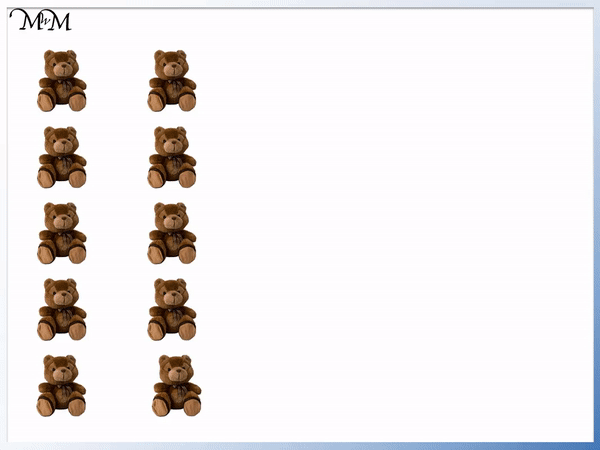• We can count the bears in ones and see that there are 10 in total.
• To count the bears more quickly, we can count them in groups of two.
• Counting in twos we have: 2, 4, 6, 8, 10.
• To count up in twos, add two each time.
• Skip counting is quicker than counting in ones.
• We only say the numbers: 2, 4, 6, 8 and 10.
• We do not say the numbers that we skip: 1, 3, 5, 7 and 9.

Skip counting is quicker than counting in ones.

To skip count by 2, add two each time to get to the next number.• To skip count by 2, add two to get to the next number.
• Starting at zero and adding two we have: 2, 4, 6 and 8.
• If we add two again we get to 10, which ends in a zero.
• Continuing to add 2 we have: 12, 14, 16 and 18.
• We can see that there is a pattern that repeats.
• The numbers end in 0, 2, 4, 6 and 8, which is a pattern that continues to repeat.

Starting from zero and skip counting in twos, there is a pattern of numbers which end in 0, 2, 4, 6 and 8.#### Skip Counting by Two: Accompanying Lesson Worksheet# Skip Counting by 2

## What is Skip Counting by 2?

Skip counting by 2 means to count up in twos rather than in ones. This is a quicker way to count because we only need to count every other number. We skip out the numbers in between.

Skip counting is important because it is faster than counting in ones and it is an important skill that helps to build a deeper understanding of multiplication, division, addition and subtraction.

We will use skip counting to help count the number of teddy bears in the introduction example below.

Counting the number of bears by counting in ones we have: 1, 2, 3, 4, 5, 6, 7, 8, 9 and 10.Instead, these bears can be counted in twos. We can pair them up and add two at a time.

Skip counting in twos we have: 2, 4, 6, 8, 10.

We only had to count five numbers: 2, 4, 6, 8 and 10.

It was faster to skip count by 2. We did not say the numbers in between: 1, 3, 5, 7 or 9.## How to Skip Count in Twos

To skip count in twos, keep adding two to get to the next number. Alternatively, simply count every other number.

To skip count by 2 from an even number, repeat the digits 0, 2, 4, 6, 8 and increase the tens digit by 1 each time.

To skip count by 2 from an odd number, repeat the digits 1, 3, 5, 7, 9 and increase the tens digit by 1 with each cycle.

When skip counting by 2 from zero we can notice a pattern.

We have the numbers: 0, 2, 4, 6, 8

and then: 10, 12, 14, 16, 18We can see that the numbers end in the digits: 0, 2, 4, 6, 8, which is a pattern which repeats over and over again.The 0, 2, 4, 6, 8 digit pattern repeats once it reaches the end.

Once we get to 8 and add two, we get to 10. 10 ends in 0, which is back to the start of the 0, 2, 4, 6, 8 sequence.We can see that the pattern of 0, 2, 4, 6, 8 digits is repeated but with 10 added to every number.

To continue skip counting by 2, simply repeat the 0, 2, 4, 6, 8 pattern, increasing the tens digit by 1 each time.

So we have 0, 2, 4, 6, 8 with a ‘1’ digit in front to show that we have added a ten.

We have 10, 12, 14, 16, 18.

Then we repeat 0, 2, 4, 6 8 but with a ‘2’ in front.

We have 20, 22, 24, 26, 28.

We then will have a ‘3’ in the tens and the 0, 2, 4, 6, 8 pattern in the units.

We have 30, 32, 34, 36, 38.

We can continue this pattern with ‘4’ in the tens, ‘5’ in the tens etc.

If we started at one instead of zero, then we see a different pattern.

Here is how to skip count in twos with odd numbers. To skip count in twos with odd numbers, we simply say each odd number and miss out the even numbers.

1 + 2 = 3

3 + 2 = 5

5 + 2 = 7

7 + 2 = 9

We can see that we have the pattern of digits: 1, 3, 5, 7, 9.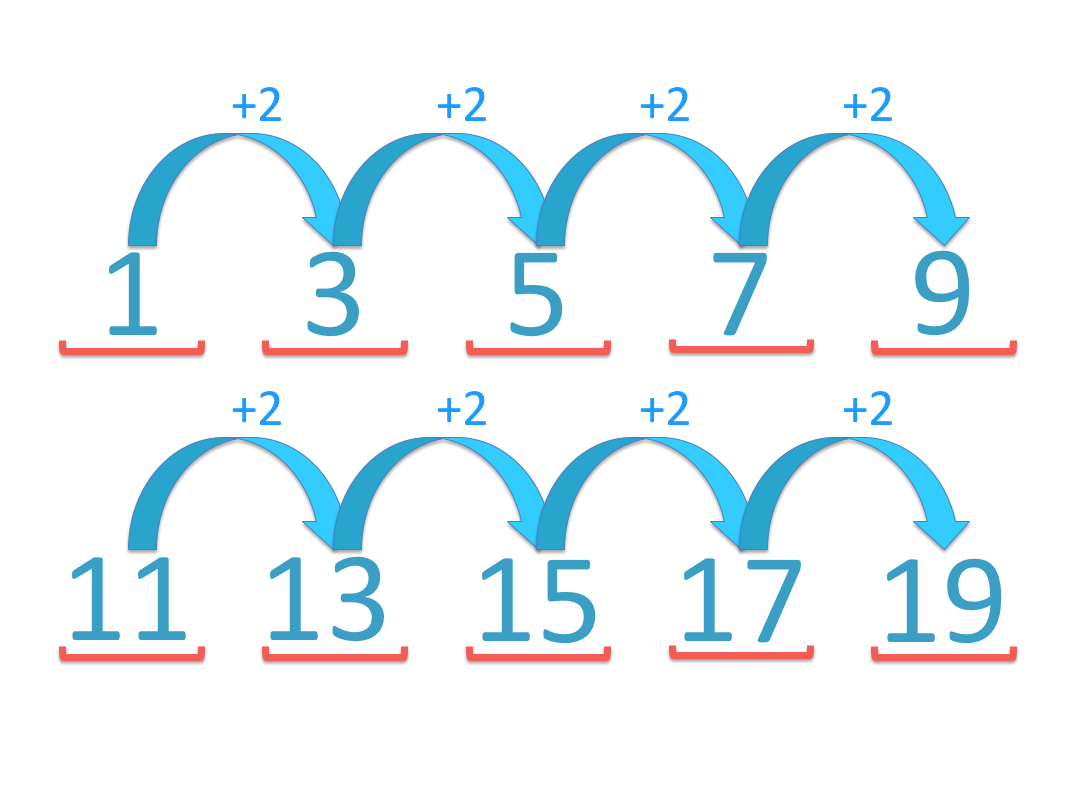Continuing to add 2, we have: 11, 13, 15, 17, 19.

We can see that these numbers end in the digits: 1, 3, 5, 7 and 9, just like the first row of numbers.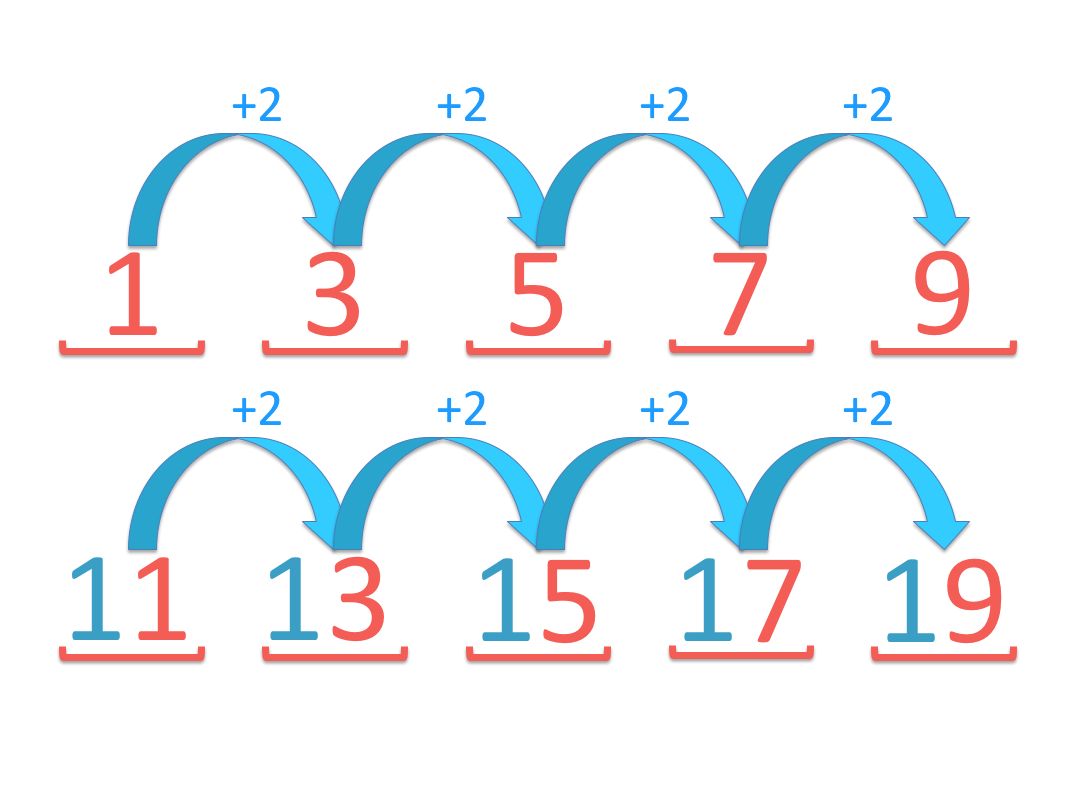This is the alternative pattern that we will see with skip counting by 2.

If we start with an odd number then we have the pattern: 1, 3, 5, 7, 9 in the digits.

The tens digit will increase by 1 each time we cycle through this pattern of digits.

We will see the 1, 3, 5, 7, 9 pattern with a ‘2’ digit in front in the tens column.

This will be 21, 23, 25, 27, 29.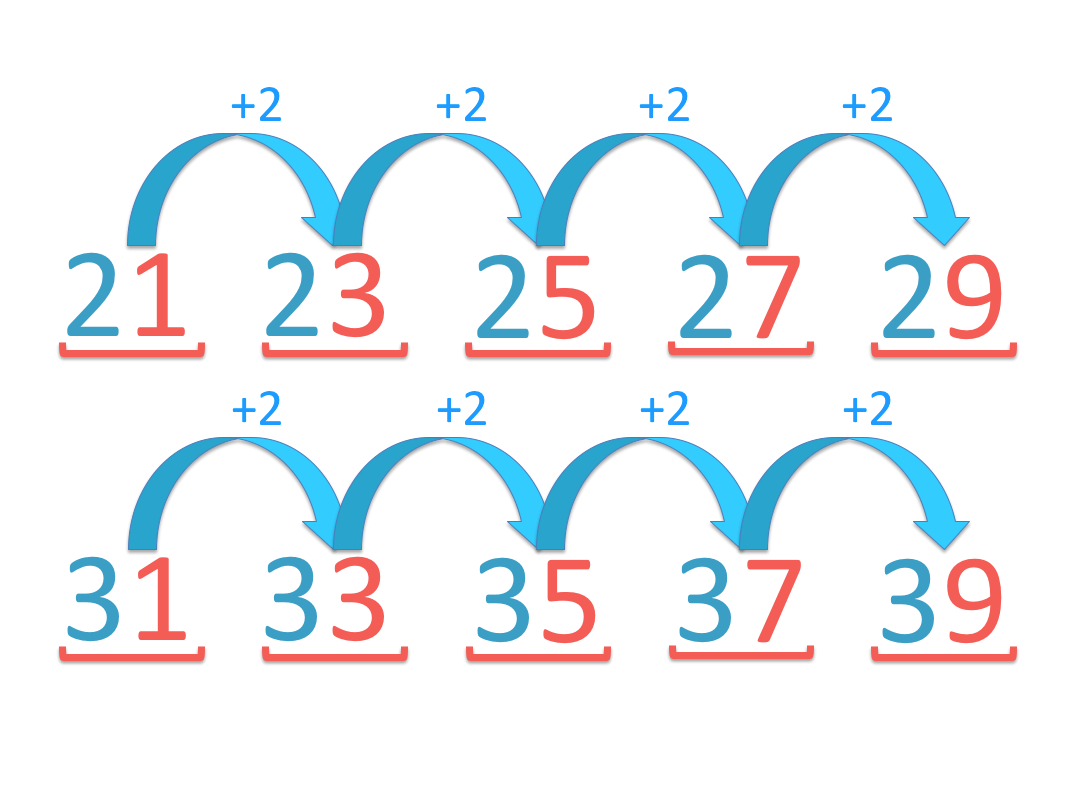After this we have the 1, 3, 5, 7, 9 pattern with a ‘3’ in the tens digit in front.

This is 31, 33, 35, 37, 39.

This pattern will continue on with 41, 43, 45, 47, 49 and then 51, 53, 55, 57, 59, then 61, 63, 65, 67, 69 and so on.

## How to Teach Skip Counting by 2

To Teach skip counting by 2, it is useful to start by using real-life objects and grouping them into pairs. Start by counting up from zero in ones but do not say the odd numbers. Eventually move on to starting at numbers that are larger than zero and odd numbers.

For example, we will introduce skip counting by twos with counting the number of teddy bears here.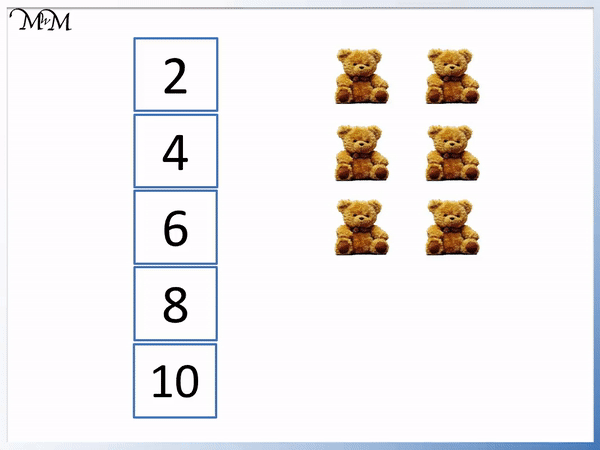Starting from zero and adding two each time we have: 2, 4, 6.The general idea behind skip counting by 2 is to only say every other number. Once this is understood, it is helpful to practise skip counting with larger numbers. Lots of practice is recommended and skip counting worksheets like the ones below are useful.

Below is a skip counting caterpillar that we can fill with numbers by skip counting in twos.We can complete the skip counting caterpillar by adding 2 to each number. Since we are skip counting in twos from zero, we will have the 0, 2, 4, 6, 8 pattern repeating with each tens digit.Here is the completed skip counting caterpillar worksheet answers.When teaching skip counting, it is important to pick out patterns early on and use these to help memorise the numbers.

Children will enjoy picking out the repeating pattern and it can be helpful to chant or sing the patterns as you teach the numbers. We can also use number grids to show the patterns and you can point at each number as it comes up.

It is also helpful to break down the patterns, teaching a string of numbers at a time, pausing and then repeating the string of numbers. For example, we can count 20, 22, 24, 26, 28, then pause and then count 30, 32, 34, 36, 38, pause then count 40, 42, 44, 46, 48 and so on.

## Skip Counting by 2 Backwards

To skip count backwards by 2, simply subtract 2 each time to get to the next number. Skip counting backwards in twos is like counting backwards in ones but only saying every other number. It is easiest to learn how to skip count forwards in twos before learning to skip count backwards.

We will now look at skip counting backwards by 2.

We will have the same number pattern as before but we will be subtracting 2 each time as we go backwards.

When teaching skip counting, it is easiest to teach skip counting forwards first and then introduce skip counting backwards as a way to practise using the exact same number patterns.

In this example, we will have the number pattern 0, 2, 4, 6, 8 but backwards.

Starting at 8, we will then have 6, 4, 2, 0.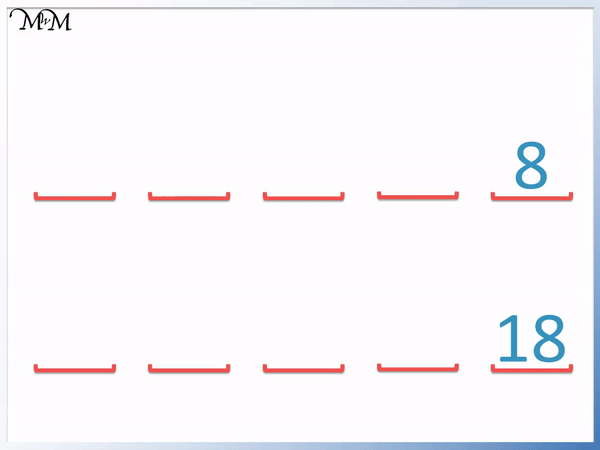We will have the same pattern of 8, 6, 4, 2, 0 when we count back from 18.

We have: 18, 16, 14, 12, 10.

We can see the digits repeat this pattern.We will now practise skip counting by 2 backwards using a skip counting caterpillar worksheet.

Below is a blank skip counting caterpillar that we can use to count backwards from 60.

We will take away two each time we skip count backwards.Here is the example of the skip counting caterpillar worksheet being completed.Here are the answers to the skip counting caterpillar worksheet.To skip count in twos from zero, the digits in the units column will move from 0, 2, 4, 6, 8 and repeat with each new tens digit.

To skip count backwards in twos with these same numbers, the digits will decrease in the same pattern of 8, 6, 4, 2, 0.Now try our lesson on Skip Counting by 3 where we learn tips for skip counting in threes.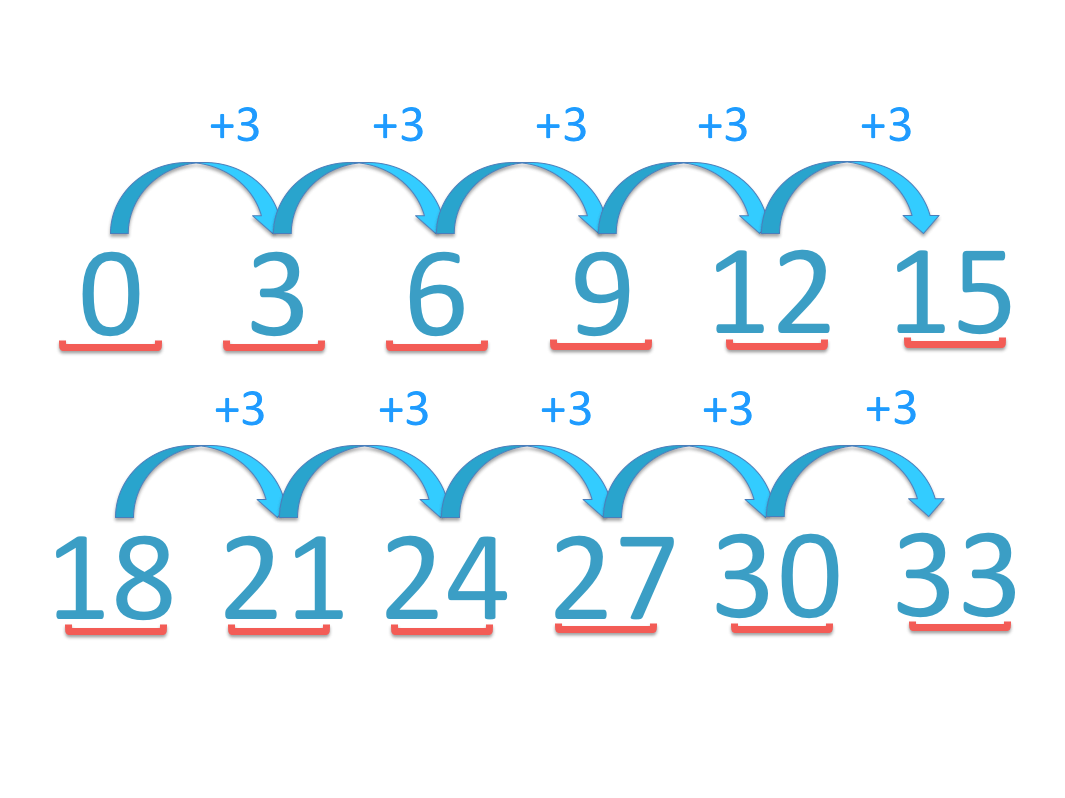error: Content is protected !!